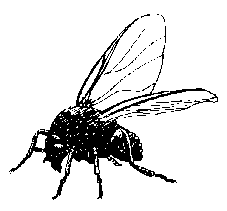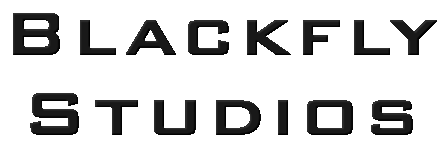#### Color to Grayscale

There are numerous methods for computing a grayscale image from a color image. One of the simplest is to average all three color channels:

``` (R + G + B)/3 ```

This is identical to applying a weight factor of 1/3 to each of the color channels:

``` 1/3R + 1/3G + 1/3B ```

However, this method tends to produce muddy images, mainly because the human eye responds to different frequencies of light differently. A better method involves adjusting the weightings to match the perceived luminosity of each color channel. There are several models for this. A commonly cited model places these values at 0.2126 for red, 0.7152 for green, and 0.0722 for blue.

``` 0.2126R + 0.7152G + 0.0722B ```

Of course, computations of this form assume a linear response curve for both the human eye and the display device, neither of which is correct. Indeed, an accurate and precise model is likely to be compuationally very expensive - which brings me to the purpose of this article...

For computational efficiency, it is imperative to avoid floating point arithmetic, and generally avoid expensive integer computations such as multiply and divide. Remembering that multiplying and dividing by powers of two can be replicated using shift operations, the following weightings can be applied which are reasonably close to the luminosity model:

``` 0.25R + 0.625G + 0.125B or (2R + 5G + B)/8 ```

This can be computed as follows:

``` ((R shl 1) + (G shl 2) + G + B) shr 3 ```

Converting this to practical assembly, assuming ESI is a 24 bit source image, and EDI is an 8 bit destination image:

``` mov AX,[ESI] movzx DX,AH //DX = Green xor AH,AH //AX = Blue add AX,DX shl DX,2 add AX,DX movzx DX,[ESI+2] //DX = Red shl DX,1 add AX,DX shr AX,3 mov [EDI],AL ```

Note that if you desire an in-place conversion, EDI is not necessary, and the last line can be replaced with:

``` mov [ESI],AL mov AH,AL mov [ESI+1],AX ```

A complete Delphi implementation of the converter:

``` procedure ColorToGray(BMP: TBitmap); var Y: integer; pSrc: PRGBTriple; pDest: PByte; W: longword; Work: TBitmap; begin BMP.PixelFormat := pf24Bit; W := BMP.Width; Work := TBitmap.Create; try Work.PixelFormat := pf8Bit; Work.Palette := GetGrayPalette; Work.SetSize(W, BMP.Height); for Y := 0 to BMP.Height-1 do begin pSrc := BMP.ScanLine[Y]; pDest := Work.ScanLine[Y]; asm push EDI push ESI mov ESI,pSrc mov EDI,pDest mov ECX,W @@Loop: mov AX,[ESI] movzx DX,AH xor AH,AH add AX,DX shl DX,2 add AX,DX movzx DX,[ESI+2] shl DX,1 add AX,DX shr AX,3 mov [EDI],AL add ESI,3 inc EDI dec ECX jnz @@Loop pop ESI pop EDI end; end; BMP.assign(Work); finally Work.Free; end; end; ```

#### Grayscale Palettes

Sometimes you don't need color, and single channel image data is enough. This is frequently true of document imaging and some types of photography like thermal and infrared. Unfortunately, Windows does not natively support one-channel images, and so a grayscale palette must be constructed to represent the image.

The following functions will produce grayscale palettes for the appropriate pixel format. The first produces the full 8-bit spectrum:

``` function GetGrayPalette: HPALETTE; var pPal: PLogPalette; begin GetMem(pPal,1028); try asm push EDI mov EDI,pPal mov [EDI],\$300 mov ECX,\$100 mov [EDI+2],CX mov EDX,\$010101 xor EAX,EAX @@Loop: add EDI,4 mov [EDI],EAX add EAX,EDX dec ECX jnz @@Loop pop EDI end; result:=CreatePalette(pPal^); finally FreeMem(pPal); end; end; ```

An evenly distributed 4-bit palette:

``` function GetGrayPalette16: HPALETTE; var pPal: PLogPalette; begin GetMem(pPal,68); try asm push EDI mov EDI,pPal mov [EDI],\$300 mov ECX,\$10 mov [EDI+2],CX mov EDX,\$111111 xor EAX,EAX @@Loop: add EDI,4 mov [EDI],EAX add EAX,EDX dec ECX jnz @@Loop pop EDI end; result:=CreatePalette(pPal^); finally FreeMem(pPal); end; end; ```

Bi-tonal images typically do not require a palette as it assumed on most devices that 0 = black and 1 = white. However, for completeness, the 1-bit black and white version:

``` function GetBWPalette: HPALETTE; var pPal: PLogPalette; begin GetMem(pPal,12); try asm mov EAX,pPal mov [EAX],\$20300 mov [EAX+4],longword(0) mov [EAX+8],\$FFFFFF end; result:=CreatePalette(pPal^); finally FreeMem(pPal); end; end;```